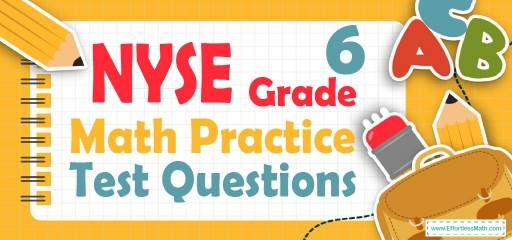# 6th Grade NYSE Math Practice Test QuestionsTherefore, in this blog post, we provide you with a collection of 10 commonly used practice questions and their step-by-step solutions to help your student prepare for the 6th Grade NYSE Math test as much as possible.

## 10 Sample 6th Grade NYSE Math Practice Questions

1- What is the missing prime factor of number 420?
$$420=2^2×3^1×…$$

A. $$2^2×3^1×5^1×7^1$$

B. $$2^2×3^1×7^1×9^1$$

C. $$1^2×2^3×2^1×3^1$$

D. $$3^2×5^1×7^1×9^1$$

2- If the area of the following trapezoid is equal to $$A$$, which equation represents $$x$$?

A. $$x = \frac{13}{A}$$

B. $$x = \frac{A}{13}$$

C. $$x=A+13$$

D. $$x=A-13$$

3- By what factor did the number below change from the first to the fourth number?
$$8, 104, 1352, 17576$$

A. 13

B. 96

C. 1456

D. 17568

4- 170 is equal to …

A. $$-20-(3×10)+(6×40)$$

B. $$((\frac{15}{8})×72 )+ (\frac{125}{5})$$

C. $$((\frac{30}{4} + \frac{15}{2})×8) – \frac{11}{2} + \frac{222}{4}$$

D. $$\frac{481}{6} + \frac{121}{3}+50$$

5- The distance between the two cities is 3,768 feet. What is the distance of the two cities in yards?

A. 1,256 yd

B. 11,304 yd

C. 45,216 yd

D. 3,768 yd

6- Mr. Jones saves $3,400 out of his monthly family income of$74,800. What fractional part of his income does Mr. Jones save?

A. $$\frac{1}{22}$$

B. $$\frac{1}{11}$$

C. $$\frac{3}{25}$$

D. $$\frac{2}{15}$$

7- What is the lowest common multiple of 12 and 20?

A. 60

B. 40

C. 20

D. 12

8- Based on the table below, which expression represents any value of f in terms of its corresponding value of $$x$$?

A. $$f=2x-\frac{3}{10}$$

B. $$f=x+\frac{3}{10}$$

C. $$f=2x+2 \frac{2}{5}$$

D. $$2x+\frac{3}{10}$$

9- 96 kg $$=$$… ?

A. 96 mg

B. 9,600 mg

C. 960,000 mg

D. 96,000,000 mg

10- Calculate the approximate area of the following circle? (the diameter is 25)

A. 78

B. 491

C. 157

D. 1963

## Best 6th Grade NYSE Math Prep Resource for 2022

1- A
$$420=2^2×3^1×5^1×7^1$$

2- B
The area of the trapezoid is: area= $$\frac{(base 1+base 2)}{2})×height= ((\frac{10 + 16}{2})x = A$$
$$→13x = A→x = \frac{A}{13}$$

3- A
$$\frac{104}{8}=13, \frac{1352}{104}=13, \frac{17576}{1352}=13$$
Therefore, the factor is 13

4- C
Simplify each option provided.
$$A. -20-(3×10)+(6×40)=-20-30+240=190$$
$$B. (\frac{15}{8})×72 + (\frac{125}{5}) =135+25=160$$
$$C. ((\frac{30}{4} + \frac{15}{2})×8) – \frac{11}{2} + \frac{222}{4} = ((\frac{30 + 30}{4})×8)- \frac{11}{2}+ \frac{111}{2}=(\frac{60}{4})×8) + \frac{100}{2}= 120 + 50 = 170$$this is the answer
$$D. \frac{481}{6} + \frac{121}{3}+50= \frac{481+242}{6}+50=120.5+50=170.5$$

5- A
1 yard $$=$$3 feet
Therefore, $$3,768 ft × \frac{1 \space yd }{3 \space ft}=1,256 \space yd$$

6- A
3,400 out of 74,800 equals to $$\frac{3,400}{74,800}=\frac{17}{374}=\frac{1}{22}$$

7- A
Prime factorizing of $$20=2×2×5$$
Prime factorizing of $$12=2×2×3$$
LCM$$=2×2×3×5=60$$

8- C
Plug in the value of $$x$$ into the function f. First, plug in 3.1 for $$x$$.
$$A. f=2x-\frac{3}{10}=2(3.1)-\frac{3}{10}=5.9≠8.6$$
$$B. f=x+\frac{3}{10}=3.1+\frac{3}{10}=3.4≠10.8$$
$$C. f=2x+2 \frac{2}{5}=2(3.1)+2 \frac{2}{5}=6.2+2.4=8.6$$
This is correct!
Plug in other values of $$x. x=4.2$$
$$f=2x+2\frac{2}{5} =2(4.2)+2.4=10.8$$
This one is also correct.
$$x=5.9$$
$$f=2x+2 \frac{2}{5}=2(5.9)+2.4=14.2$$
This one works too!
$$D. 2x+\frac{3}{10}=2(3.1)+\frac{3}{10}=6.5≠8.6$$

9- D
1 kg$$=$$ 1000 g and 1 g $$=$$ 1000 mg
96 kg$$=$$ 96 $$×$$ 1000 g $$=$$96 $$×$$ 1000 $$×$$ 1000 $$=$$96,000,000 mg

10- B
The diameter of a circle is twice the radius. Radius of the circle is $$\frac{25}{2}$$.
Area of a circle = $$πr^2=π(\frac{25}{2})^2=156.25π=156.25×3.14=490.625≅491$$

Looking for the best resource to help you succeed on the NYSE Math Grade 6 Math test?